##### The Japanese Bonsai specialist
Direct order Contact Help / Services Newsletter# Kiku bachi granite basin ø 60cm

Kiku bachi tsukubai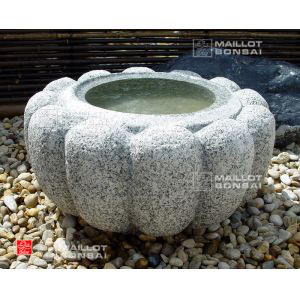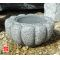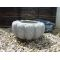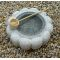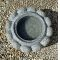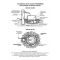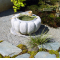ref. : 6555

350,00

voluminous/heavy item extra shipping of 55,00

Available quantity : 4Order

###### Description

Diameter 60 cm. Height 32 cm, weight 100 kilograms. Central hollow: 300 Ø * 130 mm deep. An elegant chrysanthemum-shaped kiku bachi bowl made of real granite. A bamboo water fountain or ladle makes a decorative addition.

#bachi 5.5 #basin 4.5 #granite 3.7 #water 3.5 #kiku 3.4 #tsukuba 2.7 #mizu 2.4 #chrysanthemum 2.3 #decorative 2 #kilograms 1.9

Formule
(( ROUND((CHAR_LENGTH(b.article_nom)-CHAR_LENGTH(REPLACE(b.article_nom, 'granite', '')))/LENGTH('granite')) + ROUND((CHAR_LENGTH(b.article_description)-CHAR_LENGTH(REPLACE(b.article_description, 'granite', '')))/LENGTH('granite')) ) * 3.7) + (( ROUND((CHAR_LENGTH(b.article_nom)-CHAR_LENGTH(REPLACE(b.article_nom, 'bachi', '')))/LENGTH('bachi')) + ROUND((CHAR_LENGTH(b.article_description)-CHAR_LENGTH(REPLACE(b.article_description, 'bachi', '')))/LENGTH('bachi')) ) * 3.5) + (( ROUND((CHAR_LENGTH(b.article_nom)-CHAR_LENGTH(REPLACE(b.article_nom, 'kiku', '')))/LENGTH('kiku')) + ROUND((CHAR_LENGTH(b.article_description)-CHAR_LENGTH(REPLACE(b.article_description, 'kiku', '')))/LENGTH('kiku')) ) * 3.4) + (( ROUND((CHAR_LENGTH(b.article_nom)-CHAR_LENGTH(REPLACE(b.article_nom, 'basin', '')))/LENGTH('basin')) + ROUND((CHAR_LENGTH(b.article_description)-CHAR_LENGTH(REPLACE(b.article_description, 'basin', '')))/LENGTH('basin')) ) * 2.5) + (( ROUND((CHAR_LENGTH(b.article_nom)-CHAR_LENGTH(REPLACE(b.article_nom, 'chrysanthemum', '')))/LENGTH('chrysanthemum')) + ROUND((CHAR_LENGTH(b.article_description)-CHAR_LENGTH(REPLACE(b.article_description, 'chrysanthemum', '')))/LENGTH('chrysanthemum')) ) * 2.3) + (( ROUND((CHAR_LENGTH(b.article_nom)-CHAR_LENGTH(REPLACE(b.article_nom, 'decorative', '')))/LENGTH('decorative')) + ROUND((CHAR_LENGTH(b.article_description)-CHAR_LENGTH(REPLACE(b.article_description, 'decorative', '')))/LENGTH('decorative')) ) * 2) + (( ROUND((CHAR_LENGTH(b.article_nom)-CHAR_LENGTH(REPLACE(b.article_nom, 'kilograms', '')))/LENGTH('kilograms')) + ROUND((CHAR_LENGTH(b.article_description)-CHAR_LENGTH(REPLACE(b.article_description, 'kilograms', '')))/LENGTH('kilograms')) ) * 1.9) + (( ROUND((CHAR_LENGTH(b.article_nom)-CHAR_LENGTH(REPLACE(b.article_nom, 'fountain', '')))/LENGTH('fountain')) + ROUND((CHAR_LENGTH(b.article_description)-CHAR_LENGTH(REPLACE(b.article_description, 'fountain', '')))/LENGTH('fountain')) ) * 1.8) + (( ROUND((CHAR_LENGTH(b.article_nom)-CHAR_LENGTH(REPLACE(b.article_nom, 'addition', '')))/LENGTH('addition')) + ROUND((CHAR_LENGTH(b.article_description)-CHAR_LENGTH(REPLACE(b.article_description, 'addition', '')))/LENGTH('addition')) ) * 1.8) + (( ROUND((CHAR_LENGTH(b.article_nom)-CHAR_LENGTH(REPLACE(b.article_nom, 'diameter', '')))/LENGTH('diameter')) + ROUND((CHAR_LENGTH(b.article_description)-CHAR_LENGTH(REPLACE(b.article_description, 'diameter', '')))/LENGTH('diameter')) ) * 1.8)

## Secure payment## Delivery

Our logistic partners :04 74 55 23 48
Pépinière MAILLOT-BONSAÏ
Le Bois Frazy
01990 RELEVANT - FRANCE
on appointment